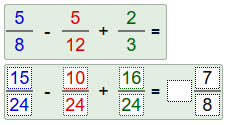Add and subtractunlike fractions Determine the common denominator. Add or subtract the numerators of the fractions. Write the result as a mixed number and reduce! Example:Click on  new  to create a new problem.
Can you top 249 points?

 Expand the three fractions and write the result as a mixed number!
 =
 =

realmath.de

... more than just practicing